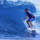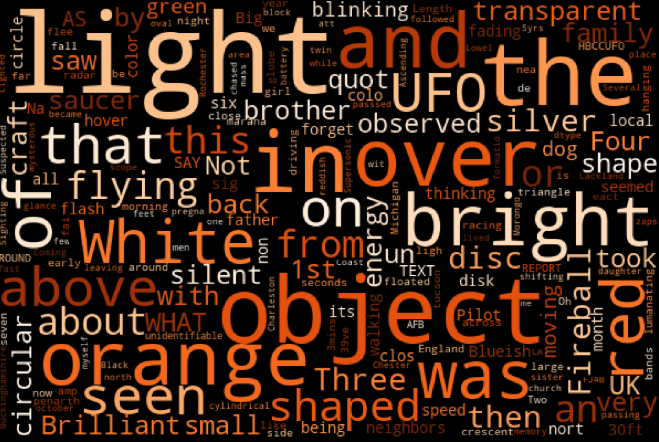# Visualizing Text Data With WordClouds

A popular way of visualizing text data is using WordClouds. Using Python's WordCloud library this can be done with a column of text data and a few lines of code.

```# SQL output is imported as a pandas dataframe variable called "df"
import matplotlib.pyplot as plt
from wordcloud import WordCloud, STOPWORDS

stopwords = ["my","to","at","for"] # removes any words you may want to exclude from your wordcloud

img = WordCloud(width = 600, height = 400,
background_color='black', colormap = 'Oranges',
stopwords=stopwords,
max_words=200,
max_font_size=100,
random_state=42
).generate(str(df["TEXT"]))

plt.figure(figsize=(30,20))
plt.subplots_adjust(left=0, right=1, top=1, bottom=0)
plt.imshow(img)
plt.axis('off')
periscope.output(plt)```

Here is our result!